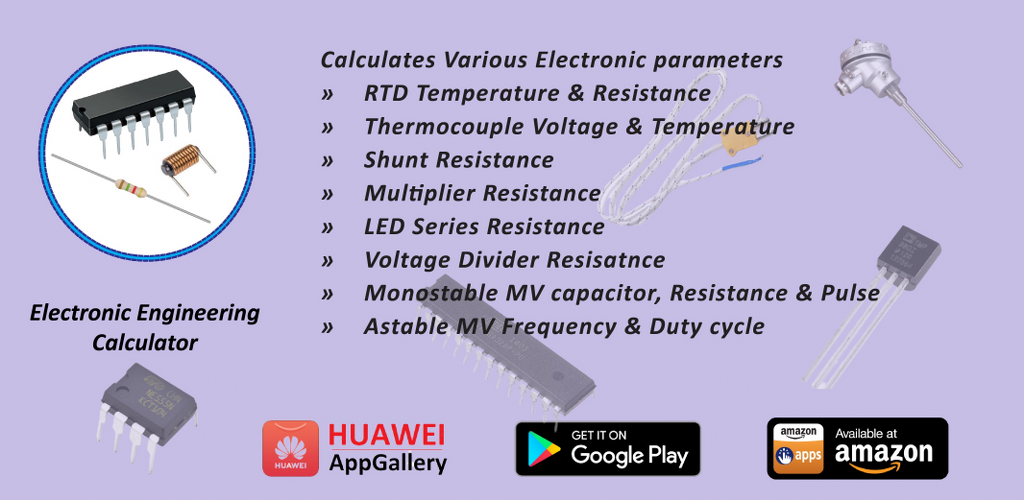# Electronic Engineering Calculator

It calculates various electronic parameters used for engineering Students, Hobbyist & Professionals in daily use. Currently it supports RTD Resistance & temperature calculation, Thermistor Resistance and temperature calculation,Thermocouple voltage & Temperature calculation, LM34 & 35 temperature & voltage, Shunt Resistance, Multiplier resistance, Voltage divider resistance, LED series resistance, Astable Multivibrator frequency and Duty cycle, Monostable Resistance, capacitance & Pulse calculation, OP-AMP Gain Calculation, Zener diode resistance and power calculation, LM317T calculator, mA to Process variable(PV) and PV to mA converter.